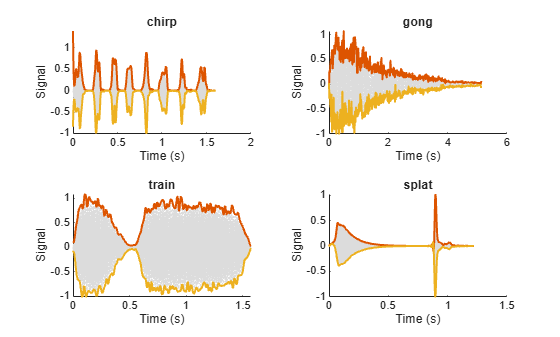# combine

Combine data from multiple datastores

Since R2020a

## Syntax

``sdsnew = combine(sds1,sds2,...,sdsn)``

## Description

example

````sdsnew = combine(sds1,sds2,...,sdsn)` combines two or more datastores by horizontally concatenating the data returned by the `read` function called on the input datastores.```

## Examples

collapse all

Specify the path to four signals included with MATLAB®. The signals are recordings of a bird chirp, a gong, a train, and a splat. All signals are sampled at 8192 Hz.

```folder = fullfile(matlabroot,'toolbox','matlab','audiovideo', ... ["chirp.mat","gong.mat","train.mat","splat.mat"]);```

Create a signal datastore that points to the specified files. Each file contains the variable `Fs` that denotes the sample rate.

`sds1 = signalDatastore(folder,'SampleRateVariableName','Fs');`

Define a function that takes the output of the `read` function and calculates the upper and lower envelopes of the signals using spline interpolation over local maxima separated by at least 80 samples. The function also returns the sample times for each signal.

```function [dataOut,infoOut] = signalEnvelope(dataIn,info) [dataOut(:,1),dataOut(:,2)] = envelope(dataIn,80,'peak'); infoOut = info; infoOut.TimeInstants = (0:length(dataOut)-1)/info.SampleRate; end ```

Call the `transform` function to create a second datastore, `sds2`, that computes the envelopes of the signals using the function you defined.

`sds2 = transform(sds1,@signalEnvelope,"IncludeInfo",true);`

Combine `sds1` and `sds2` create a third datastore. Each call to the `read` function from the combined datastore returns a matrix with three columns:

• The first column corresponds to the original signal.

• The second and third columns correspond to the upper and lower envelopes, respectively.

`sdsCombined = combine(sds1,sds2);`

Read and display the original data and the upper and lower envelopes from the combined datastore. Use the `extractBetween` function to extract the file name from the file path.

```tiledlayout('flow') while hasdata(sdsCombined) [dataOut,infoOut] = read(sdsCombined); ts = infoOut{2}.TimeInstants; nexttile hold on plot(ts,dataOut(:,1),'Color','#DCDCDC','LineStyle',':') plot(ts,dataOut(:,2:3),'Linewidth',1.5) hold off xlabel('Time (s)') ylabel('Signal') title(extractBetween(infoOut{:,2}.FileName,'audiovideo\','.mat')) end```Each file in the `sample_chirps` folder contains a chirp and a random sample rate ranging from 100 to 150 Hz. Create a signal datastore that points to the specified folder and set the name of the sample rate variable.

```folder = "sample_chirps"; sds = signalDatastore(folder,SampleRateVariableName="fs");```

Define a function that takes the output of the `read` function and uses the `pspectrum` function to estimate the power spectrum of the signal. Use the estimate to compute the instantaneous frequency. The function also returns the vector of time instants corresponding to the centers of the windowed segments and the frequencies corresponding to the spectral estimates contained in the spectrograms of the signals.

```function [dataOut,infoOut] = extractinstfreq(dataIn,info) [P,F,T] = pspectrum(dataIn,info.SampleRate,"spectrogram",... TimeResolution=0.1,OverlapPercent=40,Leakage=0.8); dataOut = {instfreq(P,F,T)'}; infoOut = info; infoOut.CenterFrequencies = F; infoOut.TimeInstants = T; end ```

Call the `transform` function to create a new datastore that computes the instantaneous frequencies.

`sds2 = transform(sds,@extractinstfreq,IncludeInfo=true);`

Because the data in `sds2` is not horizontally concatenable with the data in `sds`, transform the data in `sds` into cell arrays.

`sds1 = transform(sds,@(x) {x});`

Combine `sds1` and `sds2`. While the combined datastore has unread files, read from the new datastore and visualize the spectrograms. Overlay the instantaneous frequencies on the spectrograms.

```sdsCombined = combine(sds1,sds2); sdsSubset = subset(sdsCombined,[1,4,9,10]); plotID = 1; while hasdata(sdsSubset) subplot(2,2,plotID) [sig,info] = read(sdsSubset); pspectrum(sig{:,1},info{:,2}.SampleRate,"spectrogram", ... TimeResolution=0.1,OverlapPercent=40,Leakage=0.8) hold on plot(info{:,2}.TimeInstants',sig{:,2}) plotID = plotID + 1; end```## Input Arguments

collapse all

Signal datastores to combine, specified as two or more comma-separated `signalDatastore` objects.

## Output Arguments

collapse all

New datastore with the combined data, returned as a `CombinedDatastore` object.

Calling `read` on the combined datastore, horizontally concatenates the data by calling `read` on each input datastore.

## Version History

Introduced in R2020a